Electrical Dost

What is insulation resistance?What is insulation resistance? Obtain an expression for insulation resistance of single corecable. What is the effect of temperature and moisture on the resistance of an insulating material?

Opposition offered by the insulating material to the flow of leakage current is called insulation
resistance.

Expression Of Insulation Resistance:

Consider a cable of length l (m). If we cut a cable, cross section of cable will look as shown in
the figure.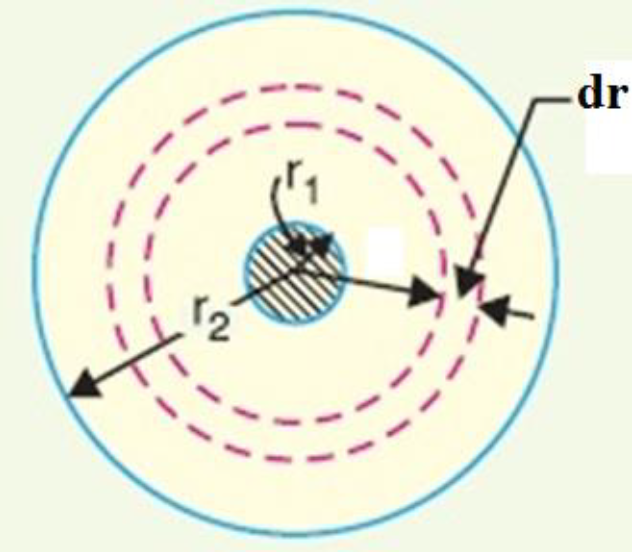Let, l = length of cable in m

Thickness of insulation = r = r2 – r1

Let, dr be the small section of thickness.

Let, dri be the insulation resistance offered by dr.

The insulation resistance –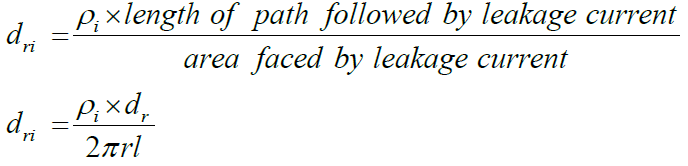Total insulation resistance is,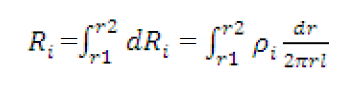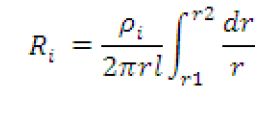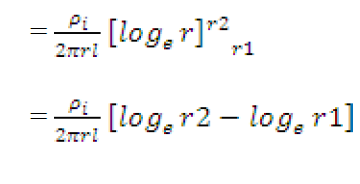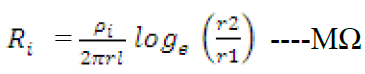Effect of Temperature & Moisture on Insulation Resistance:

When temperature increases, the valance electrons which are loosely bound to the nucleus, acquire thermal energy. Due to the additional energy acquired, the valance electrons become completely free from the force of attraction by the nucleus. These electrons are available as the free electrons. More number of free electrons, better is the conductivity and less is the resistivity. Hence as temperature increases, the insulation resistance decreases while conductivity increases.
The effect can be mathematically expressed interms of an exponential relationship as,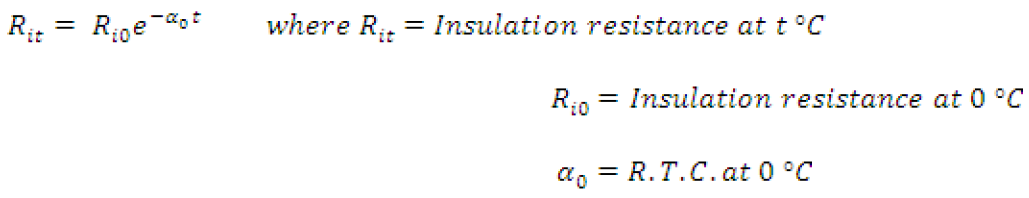Practically insulation covering the conductor may absorb some moisture. As water is good conductor of electricity, hence possibility of conduction increases through the insulation when it absorb water. As moisture content in insulation resistance increases, the insulation resistance decreases.

Krushna Bochare

Electrical Engineer, Content Creator, Working At PowerIT Solutions Pvt Ltd.

Related Posts

What is the operating principle of a single-phase transformer?

Single-phase transformer Introduction: The transformer is a static device (i.e. the one which does not contain any rotating or moving parts) which is used to